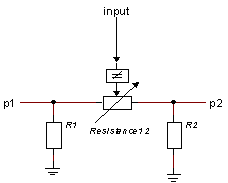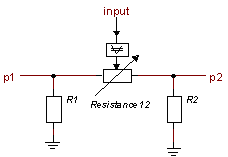﻿ 20-sim webhelp > Library > Iconic Diagrams > Electric > Components > Switch

# Switch

## Library

Iconic Diagrams\Electric\Components

Level

Amplitude

## Use

Domains: Continuous. Size: 1-D. Kind: Iconic Diagrams (Electric).

## Description - Level

This model represents an almost ideal switch. The switch is turned on when the input signal is larger than the threshold value vt and it is turned off when the input signal is smaller or equal to the threshold value vt.

The heart of the model consist of a resistance that can be changed by an input signal from almost zero (on) to a very large value (off). By proper selection of the on and off resistances, they can be effectively zero and infinity in comparison to other circuit elements.Layout of the switch model.

The equations of the resistance are:

R = if input > vt then Ron else Roff end;

p.u = R * p.i;

## Interface - Level

 Ports Description p1, p2 input Both electric ports (Electric). The switching signal. Causality indifferent p1, p2 Parameters R1\R R2\R Resistance12\Ron Resistance12\Roff Resistance12\vt Ground resistance at port 1 [Ohm] Ground resistance at port 2 [Ohm] Resistance when switch is turned on [Ohm] Resistance when switch is turned off [Ohm] Threshold value, switch is turned on when input > vt

## Description - Amplitude

This model represents an almost ideal switch. The switch is turned on when the amplitude of the input signal is larger than the threshold value vt and it is turned off when the amplitude of the input signal is smaller or equal to the threshold value vt.

The heart of the model consist of a resistance that can be changed by an input signal from almost zero (on) to a very large value (off). By proper selection of the on and off resistances, they can be effectively zero and infinity in comparison to other circuit elements.Layout of the switch model.

The equations of the resistance are:

R = if abs(input) > vt then Ron else Roff end;

p.u = R * p.i;

## Interface - Amplitude

 Ports Description p1, p2 input Both electric ports (Electric). The switching signal. Causality indifferent p1,p2 Parameters R1\R R2\R Resistance12\Ron Resistance12\Roff Resistance12\vt Ground resistance at port 1 [Ohm] Ground resistance at port 2 [Ohm] Resistance when switch is turned on [Ohm] Resistance when switch is turned off [Ohm] Threshold value, switch is turned on when abs(input) > vt